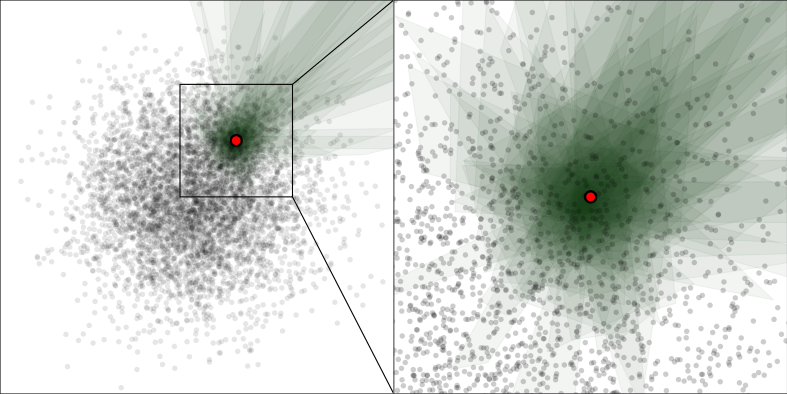# MRPT - fast nearest neighbor search with random projectionMRPT is a lightweight and easy-to-use library for approximate nearest neighbor search. It is written in C++11 and has Python bindings. The index building has an integrated hyperparameter tuning algorithm, so the only hyperparameter required to construct the index is the target recall level!

According to our experiments MRPT is one of the fastest libraries for approximate nearest neighbor search.

In the offline phase of the algorithm MRPT indexes the data with a collection of random projection trees. In the online phase the index structure allows us to answer queries in superior time. A detailed description of the algorithm with the time and space complexities, and the aforementioned comparisons can be found in our article that was published in IEEE International Conference on Big Data 2016.

The algorithm for automatic hyperparameter tuning is described in detail in our new article that will be presented in Pacific-Asia Conference on Knowledge Discovery and Data Mining 2019 (arxiv preprint).

Currently the Euclidean distance is supported as a distance metric.

The tests for MRPT are in a separate repo.

## New

• Release MRPT 1.1.1 : faster autotuning and bug fixes. (2018/12/07)

• Release MRPT 1.1.0 : now autotuning works also without a separate set of test queries. (2018/11/24)

• Release MRPT 1.0.0 (2018/11/22)

• Add documentation for C++ API (2018/11/22)

• Add index building with autotuning: no more manual hyperparameter tuning! (2018/11/21)

## Python installation

C++ compiler is needed for building python wrapper.

On MacOS, LLVM is needed for compiling: `brew install llvm libomp`.

On Windows, you may use MSVC compiler.

Install the module with `pip install git+https://github.com/vioshyvo/mrpt/`

### Docker

An example docker file is provided, which builds MRPT python wrapper in Linux environment.

```docker build -t mrpt .
docker run --rm -it mrpt
```

## Minimal examples

### Python

This example first generates a 200-dimensional data set of 10000 points, and 100 test query points. The `exact_search` function can be used to find the indices of the true 10 nearest neighbors of the first test query.

The `build_autotune_sample` function then builds an index for approximate k-nn search; it uses automatic parameter tuning, so only the target recall level (90% in this example) and the number of neighbors searched for have to be specified.

```import mrpt
import numpy as np

n, d, k = 10000, 200, 10
target_recall = 0.9

data = np.random.rand(n, d).astype(np.float32)
q = np.random.rand(d).astype(np.float32)

index = mrpt.MRPTIndex(data)
print(index.exact_search(q, k, return_distances=False))

index.build_autotune_sample(target_recall, k)
print(index.ann(q, return_distances=False))
```

The approximate nearest neighbors are then searched by the function `ann`; because the index was autotuned, no other arguments than the query point are required.

Here is a sample output:

``````[9738 5033 6520 2108 9216 9164  112 1442 1871 8020]
[9738 5033 6520 2108 9216 9164  112 1442 1871 6789]
``````

### C++

MRPT is a header-only library, so no compilation is required: just include the header `cpp/Mrpt.h`. The only dependency is the Eigen linear algebra library (Eigen 3.3.5 is bundled in `cpp/lib`), so when using g++, the following minimal example can be compiled for example as:

``````g++ -std=c++11 -Ofast -march=native -Icpp -Icpp/lib ex1.cpp -o ex1 -fopenmp -lgomp
``````

Let's first generate a 200-dimensional data set of 10000 points, and a query point (row = dimension, column = data point). Then `Mrpt::exact_knn` can be used to find the indices of the true 10 nearest neighbors of the test query.

The `grow_autotune` function builds an index for approximate k-nn search; it uses automatic parameter tuning, so only the target recall level (90% in this example), and the number of neighbors searched for have to be specified. This version automatically samples a test set of 100 query points from the data set to tune the parameters, so no separate test set is required.

```#include <iostream>
#include "Eigen/Dense"
#include "Mrpt.h"

int main() {
int n = 10000, d = 200, k = 10;
double target_recall = 0.9;
Eigen::MatrixXf X = Eigen::MatrixXf::Random(d, n);
Eigen::MatrixXf q = Eigen::VectorXf::Random(d);

Eigen::VectorXi indices(k), indices_exact(k);

Mrpt::exact_knn(q, X, k, indices_exact.data());
std::cout << indices_exact.transpose() << std::endl;

Mrpt mrpt(X);
mrpt.grow_autotune(target_recall, k);

mrpt.query(q, indices.data());
std::cout << indices.transpose() << std::endl;
}
```

The approximate nearest neighbors are then searched by the function `query`; because the index was autotuned, no other arguments than a query point and an output buffer for indices are required.

Here is a sample output:

``````8108 1465 6963 2165   83 5900  662 8112 3592 5505
8108 1465 6963 2165   83 5900 8112 3592 5505 7992
``````

The approximate nearest neighbor search found 9 of 10 true nearest neighbors; so this time the observed recall happened to match the expected recall exactly (results vary between the runs because the algorithm is randomized).

## Citation

Automatic hyperparameter tuning:

``````@inproceedings{Jaasaari2019,
title={Efficient Autotuning of Hyperparameters in Approximate Nearest Neighbor Search},
author={J{\"a}{\"a}saari, Elias and Hyv{\"o}nen, Ville and Roos, Teemu},
booktitle={Pacific-Asia Conference on Knowledge Discovery and Data Mining},
pages={In press},
year={2019},
organization={Springer}
}
``````

MRPT algorithm:

``````@inproceedings{Hyvonen2016,
title={Fast nearest neighbor search through sparse random projections and voting},
author={Hyv{\"o}nen, Ville and Pitk{\"a}nen, Teemu and Tasoulis, Sotiris and J{\"a}{\"a}saari, Elias and Tuomainen, Risto and Wang, Liang and Corander, Jukka and Roos, Teemu},
booktitle={Big Data (Big Data), 2016 IEEE International Conference on},
pages={881--888},
year={2016},
organization={IEEE}
}
``````

## License

MRPT is available under the MIT License (see LICENSE.txt). Note that third-party libraries in the cpp/lib folder may be distributed under other open source licenses. The Eigen library is licensed under the MPL2.

Get A Weekly Email With Trending Projects For These Topics
No Spam. Unsubscribe easily at any time.
c-plus-plus (17,955
nearest-neighbor-search (27
approximate-nearest-neighbor-search (18
similarity-search (16